# MU puzzle facts for kids

Kids Encyclopedia Facts

The MU puzzle is a puzzle stated by Douglas Hofstadter and found in Gödel, Escher, Bach involving a simple formal system called "MIU". Hofstadter's motivation is to contrast reasoning within a formal system (ie., deriving theorems) against reasoning about the formal system itself. MIU is an example of a Post canonical system and can be reformulated as a string rewriting system.

## The puzzle

Suppose there are the symbols M, I, and U which can be combined to produce strings of symbols. The MU puzzle asks one to start with the "axiomatic" string MI and transform it into the string MU using in each step one of the following transformation rules:

 Nr. Formal rule Informal explanation Example 1. xI → xIU Add a U to the end of any string ending in I MI to MIU 2. Mx → Mxx Double the string after the M MIU to MIUIU 3. xIIIy → xUy Replace any III with a U MUIIIU to MUUU 4. xUUy → xy Remove any UU MUUU to MU

## Solution

The puzzle cannot be solved: it is impossible to change the string MI into MU by repeatedly applying the given rules. In other words, MU is not a theorem of the MIU formal system. To prove this, one must step "outside" the formal system itself.

In order to prove assertions like this, it is often beneficial to look for an invariant; that is, some quantity or property that doesn't change while applying the rules.

In this case, one can look at the total number of I in a string. Only the second and third rules change this number. In particular, rule two will double it while rule three will reduce it by 3. Now, the invariant property is that the number of Is is not divisible by 3:

• In the beginning, the number of Is is 1 which is not divisible by 3.
• Doubling a number that is not divisible by 3 does not make it divisible by 3.
• Subtracting 3 from a number that is not divisible by 3 does not make it divisible by 3 either.

Thus, the goal of MU with zero I cannot be achieved because 0 is divisible by 3.

In the language of modular arithmetic, the number n of I obeys the congruence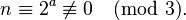$n \equiv 2^a \not\equiv 0 \pmod 3.\,$

where a counts how often the second rule is applied.

### A decidable criterion for derivability

More generally, an arbitrarily given string x can be derived from MI by the above four rules if, and only if, x respects the three following properties:

1. x is only composed with one M and any number of I and U,
2. x begins with M, and
3. the number of I in x is not divisible by 3.

#### Proof

Only if: No rule moves the M, changes the number of M, or introduces any character out of M, I, U. Therefore, every x derived from MI respects properties 1 and 2. As shown before, it also respects property 3.

If: If x respects properties 1 to 3, let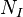$N_{I}$ and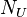$N_{U}$ be the number of I and U in x, respectively, and let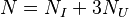$N = N_{I} + 3N_{U}$. By property 3, the number$N_{I}$ cannot be divisible by 3, hence,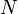$N$ cannot be, either. That is,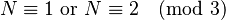$N \equiv 1 \text{ or } N \equiv 2 \pmod 3$. Let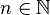$n \in \N$ such that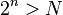$2^n > N$ and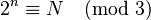$2^n \equiv N \pmod 3$. Beginning from the axiom MI, applying the second rule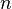$n$ times obtains MIII...I with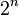$2^n$ I. Since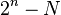$2^n - N$ is divisible by 3, by construction of$n$, applying the third rule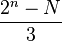$\frac{2^n - N}{3}$ times will obtain MIII...IU...U, with exactly with$N$ I, followed by some number of U. The U count can always be made even, by applying the first rule once, if necessary. Applying the fourth rule sufficiently often, all U can then be deleted, thus obtaining MIII...I with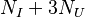$N_{I} + 3N_{U}$ I. Applying the third rule to reduce triplets of I into a U in the right spots will obtain x. Altogether, x has been derived from MI.

#### Example

To illustrate the construction in the If part of the proof, the string MIIUII, which respects properties 1 to 3, leads to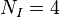$N_I=4$,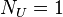$N_U=1$,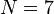$N=7$,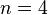$n=4$; it can be hence derived as follows:

MI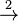$\stackrel{2}{\to}$ MII$\stackrel{2}{\to}$ MIIII$\stackrel{2}{\to}$ MIIIIIIII$\stackrel{2}{\to}$ MIIIIIIIIIIIIIIII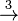$\stackrel{3}{\to}$ MIIIIIIIUIIIIII$\stackrel{3}{\to}$ MIIIIIIIUUIII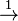$\stackrel{1}{\to}$ MIIIIIIIUUIIIU$\stackrel{3}{\to}$ MIIIIIIIUUUU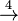$\stackrel{4}{\to}$ MIIIIIIIUU$\stackrel{4}{\to}$ MIIIIIII$\stackrel{3}{\to}$ MIIUII.

## Arithmetization

Chapter XIX of Gödel, Escher, Bach gives a mapping of the MIU system to arithmetic, as follows. First, every MIU-string can be translated to an integer by mapping the letters M, I, and U to the numbers 3, 1, and 0, respectively. (For example, the string MIUIU would be mapped to 31010.)

Second, the single axiom of the MIU system, namely the string MI, becomes the number 31.

Third, the four formal rules given above become the following:

 Nr. Formal rule Example 1. k × 10 + 1 → 10 × (k × 10 + 1) 31 → 310 (k = 3) 2. 3 × 10m + n → 10m × (3 × 10m + n) + n 310 → 31010 (m = 2, n = 10) 3. k × 10m + 3 + 111 × 10m + n → k × 10m + 1 + n 3111011 → 30011 (k = 3, m = 3, n = 11) 4. k × 10m + 2 + n → k × 10m + n 30011 → 311 (k = 3, m = 2, n = 11)

(NB: The rendering of the first rule above differs superficially from that in the book, where it is written as "[i]f we have made 10m + 1, then we can make 10 × (10m + 1)". Here, however, the variable m was reserved for use in exponents of 10 only, and therefore it was replaced by k in the first rule. Also, in this rendering, the arrangement of factors in this rule was made consistent with that of the other three rules.)

## Relationship to logic

The MIU system illustrates several important concepts in logic by means of analogy.

It can be interpreted as an analogy for a formal system — an encapsulation of mathematical and logical concepts using symbols. The MI string is akin to a single axiom, and the four transformation rules are akin to rules of inference.

The MU string and the impossibility of its derivation is then analogous to a statement of mathematical logic which cannot be proven or disproven by the formal system.

It also demonstrates the contrast between interpretation on the "syntactic" level of symbols and on the "semantic" level of meanings. On the syntactic level, there is no knowledge of the MU puzzle's insolubility. The system does not refer to anything: it is simply a game involving meaningless strings. Working within the system, an algorithm could successively generate every valid string of symbols in an attempt to generate MU, and though it would never succeed, it would search forever, never deducing that the quest was futile. For a human player however, after a number of attempts, one soon begins to suspect that the puzzle may be impossible. One then "jumps out of the system" and starts to reason about the system, rather than working within it. Eventually, one realises that the system is in some way about divisibility by three. This is the "semantic" level of the system — a level of meaning that the system naturally attains. On this level, the MU puzzle can be seen to be impossible.

The inability of the MIU system to express or deduce facts about itself, such as the inability to derive MU, is a consequence of its simplicity. However, more complex formal systems, such as systems of mathematical logic, may possess this ability. This is the key idea behind Godel's Incompleteness Theorem.

## Pedagogical uses

In her textbook, Discrete Mathematics with Applications, Susanna S. Epp uses the MU puzzle to introduce the concept of recursive definitions, and begins the relevant chapter with a quote from GEB.MU puzzle Facts for Kids. Kiddle Encyclopedia.